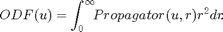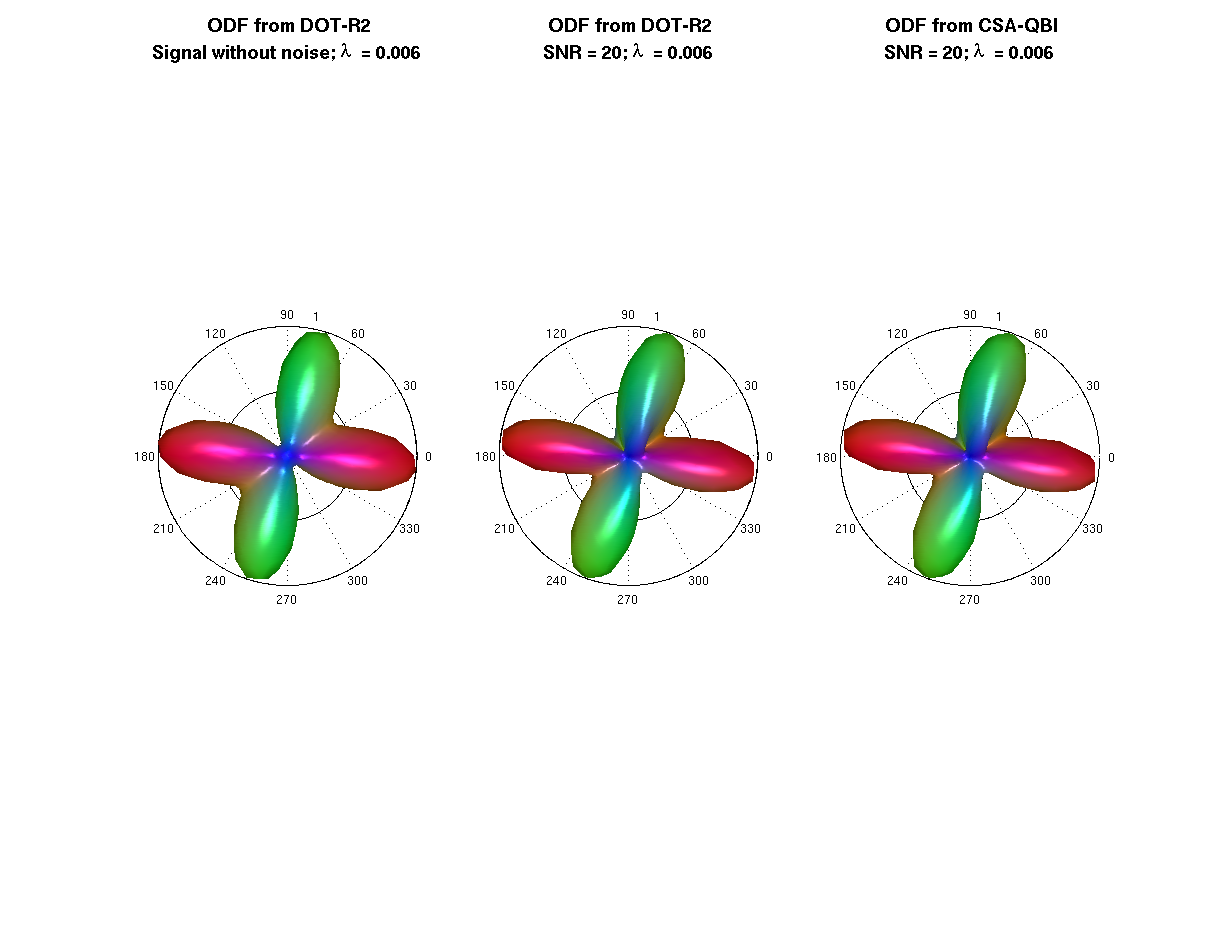# Project: High Angular Resolution Diffusion Imaging Tools

Example to illustrate the Revisited version of the Diffusion Orientation Transform (DOT) method and the Constant Solid Angle q-Ball imaging (CSA-QBI) using real spherical harmonic basis. In both methods the ODF is the radial projection of the propagator computed by the following expression:• Language: MATLAB(R)
• Author: Erick Canales-Rodríguez, Lester Melie-García, Yasser Iturria-Medina, Yasser Alemán-Gómez
• Date: 2013, Version: 1.2

## - Parameters for reconstruction: All this part is equal for each voxel

clear all; close all; clc;

% grad is a Nx3 matrix where grad(i,:) = [xi yi zi] and sqrt(xi^2 + yi^2 + zi^2) == 1 (unit vectors).
display(['The measurement scheme contains ' num2str(size(grad,1)) ' directions']);

% - Loading the reconstruction scheme (mesh where the ODF will be reconstructed)
%   ---------------------------------
% unit vectors on the sphere
% facets
display(['The reconstruction scheme contains ' num2str(size(V,1)) ' directions']);

%  --- Regularization parameter
Lambda = 0.006;
disp(' ');
display(['The regularization parameter Lambda is ' num2str(Lambda) ',']);
display ('like in the Descoteaux paper "Regularized, Fast, and Robust Analytical Q-Ball Imaging".');

% --- real spherical harmonic reconstruction: parameters definition --- %
if Lmax >= 6
Lmax = 6;
end
display(['The maximum order of the spherical harmonics decomposition is Lmax = ' num2str(Lmax) '.']);

[basisG, thetaG, phiG] = construct_SH_basis (Lmax, grad, 2, 'real');
[basisV, thetaV, phiV] = construct_SH_basis (Lmax, V, 2, 'real');
K_dot_r2 = []; Laplac2 = []; K_csa = [];

% Vector_old = [1, 3/32, 15/64, 210/512, 630/1024, 13860/16384]; % old version (There is a typo in this vector!)
% Notice that Table 1 (second row) in the article (Diffusion orientation transform revisited, Neuroimage, 2010) contains typo for L>=6:
% a term on the denominator was omitted.
% Use the following corrected result, which contains the omitted term:
% Vector = [1, 3/32, 15/64, 210/(3*512), 630/(1024*3*5), 13860/(16384*3*5*7)];
Vector = [1, 3/32, 15/64, 210/1536, 630/15360, 13860/1720320];
for L=0:2:Lmax
for m=-L:L
% -- Precomputation for DOT-R2 method
factor_dot_r2 = ((-1)^(L/2))*Vector(1,L/2 + 1)*(4/pi);
K_dot_r2 = [K_dot_r2; factor_dot_r2];
% -- Precomputation for CSA-QBI method
if L > 0
factor_csa = (-1/(8*pi))*((-1)^(L/2))*prod(1:2:(L+1))/prod(2:(L-2));
elseif L == 0
factor_csa = 1/(2*sqrt(pi));
end
K_csa = [K_csa; factor_csa];
% -- Precomputation for the spherical harmonic regularization
Laplac2 = [Laplac2; (L^2)*(L + 1)^2];
end
end
Laplac = diag(Laplac2);

% Creating the kernel for the reconstruction
A = recon_matrix(basisG,Laplac,Lambda);
% where A = inv(Ymatrix'*Ymatrix + Lambda*Laplacian)*Ymatrix';
% and Ymatrix = basisG.
% The matrix, A, is the same for all the voxels

The measurement scheme contains 70 directions
The reconstruction scheme contains 724 directions

The regularization parameter Lambda is 0.006,
like in the Descoteaux paper "Regularized, Fast, and Robust Analytical Q-Ball Imaging".
The maximum order of the spherical harmonics decomposition is Lmax = 6.


## - Simulated diffusion MRI signal using a multi-tensor model

% --- Experimental parameters --- %
% b-value
b = 4*10^3;% s/mm^2
% diffusivities
lambda1 = 1.5*10^-3;% mm^2/s
lambda2 = 0.3*10^-3;% mm^2/s
% S0 is the image at b-value = 0
S0 = 100;
% Signal to noise ratio
SNR = 20;
display(['The signal to noise ratio in this experiment is SNR = ' num2str(SNR) '.']);
% Add a measurement with b-value = 0 into the gradient matrix (standard format in which the signal is measured in the scanner)

% --- Intravoxel properties --- %
% Using a mixture of two fibers (more fibers can be used)
% volume fraction for each fiber (the sum of this elemenst is 1 by definition)
f1 = 0.5; f2 = 0.5;
% angular orientation corresponding to each fiber (main eigenvector for each diffusion tensor)
anglesF = [0  0;
70 0];
% [ang1 ang2]: -> ang1 is the rotation in degrees from x to y axis (rotation using the z-axis);
%                 ang2 is the rotation in degrees from the x-y plane to the z axis (rotation using the y axis)
% anglesF(i,:) defines the orientation of the fiber with volume fraction fi.

%  --- Computation of the diffusion signal using the above experimental parameters and intravoxel properties %
% *** Signal with realistic rician noise
S = create_signal_multi_tensor(anglesF, [f1  f2],...

% *** Ideal signal (no noise)
Snonoise = create_signal_multi_tensor(anglesF, [f1  f2],...

The signal to noise ratio in this experiment is SNR = 20.


## - Isolate the S0 component from the signal

% --- find zeros
ind_0 = find( grad_sum == 0);
S0_est = max(S(ind_0));
S(ind_0) = []; Snonoise(ind_0) = [];


## - ODF from the signal without noise

ADCnonoise = abs(-log(Snonoise/S0)/b)';
t = 20*10^-3; % s
EulerGamma = 0.577216;
Signal_profile_nonoise = EulerGamma + log(1./Dt_nonoise);

coeff0 = A*Signal_profile_nonoise(:);
coeff0 = coeff0/coeff0(1);
K(1) = (4/pi)*(2*sqrt(pi))/16;
ss0 = coeff0.*K_dot_r2;
ODFnnoise = basisV*ss0;
ODFnnoise = ODFnnoise - min(ODFnnoise);
ODFnnoise = ODFnnoise/sum(ODFnnoise); % re-normalization


## - Regularized ODF from the noised signal using DOT-R2 method

display(' ')
display('This is the real/effective time required for computing the ODF-DOTR2 at each voxel:')
tic
t = 20*10^-3; % s
EulerGamma = 0.577216;
Signal_profile = EulerGamma + log(1./Dt);
coeff = A*Signal_profile(:);
coeff = coeff/coeff(1);
K(1) = (4/pi)*(2*sqrt(pi))/16;
ss = coeff.*K_dot_r2;
ODF = basisV*ss;
ODF = ODF - min(ODF);
ODF = ODF/sum(ODF); % re-normalization
toc


This is the real/effective time required for computing the ODF-DOTR2 at each voxel:
Elapsed time is 0.000609 seconds.


## - Regularized ODF from the noised signal using CSA-QBI method

display(' ')
display('This is the real/effective time required for computing the CSA-QBI at each voxel:')
tic
Signal_profile = log(-log(S/S0_est))';
coeff = A*Signal_profile(:);
coeff(1) = 1;
% ---
ss = coeff.*K_csa;
CSA_ODF = basisV*ss;
CSA_ODF = CSA_ODF - min(CSA_ODF);
CSA_ODF = CSA_ODF/sum(CSA_ODF); % re-normalization
toc


This is the real/effective time required for computing the CSA-QBI at each voxel:
Elapsed time is 0.000270 seconds.


## - Testing the similarity between CSA-QBI and DOT-R2 methods

total_difference = sum( abs(ODF-CSA_ODF) );
display(' ')
display(['In the numerical implementation the absolute total difference between the DOT-R2 and CSA-QBI reconstuctions is: ' num2str(total_difference)]);
display('As can be seen, the DOT-R2 and CSA-QBI methods are equivalent.')
% ---


In the numerical implementation the absolute total difference between the DOT-R2 and CSA-QBI reconstuctions is: 6.167e-14
As can be seen, the DOT-R2 and CSA-QBI methods are equivalent.


## - Plots

% --- opengl('software');
feature('UseMesaSoftwareOpenGL',1);

set(gcf, 'color', 'white');
screen_size = get(0, 'ScreenSize');
f1 = figure(1); hold on;
set(f1, 'Position', [0 0 screen_size(3) screen_size(4) ] );
% -----
Origen = [0 0 0.25]; % Voxel coordinates [x y z] where the ODF will be displayed
angle = 0:.01:2*pi;
R = ones(1,length(angle));
R1 = R.*f2/f1;
subplot(1,3,1)
polarm(angle,R,'k'); hold on; polarm(angle, R1,'k');
% the ODF is scaled only for the display
plot_ODF(ODFnnoise./max(ODFnnoise), V, F, Origen);
title({'\fontsize{14} ODF from DOT-R2';...
['Signal without noise; {\lambda}  = ' num2str(Lambda)]},'FontWeight','bold');
axis equal; axis off;

subplot(1,3,2)
polarm(angle,R,'k'); hold on; polarm(angle, R1,'k');
% the ODF is scaled only for the display
plot_ODF(ODF./max(ODF),V, F, Origen);

title({'\fontsize{14} ODF from DOT-R2';...
['SNR = ' num2str(SNR) '; {\lambda}  = ' num2str(Lambda)]},'FontWeight','bold');
axis equal; axis off;

subplot(1,3,3)
polarm(angle,R,'k'); hold on; polarm(angle, R1,'k');
% the ODF is scaled only for the display
plot_ODF(CSA_ODF./max(CSA_ODF),V, F, Origen);

title({'\fontsize{14} ODF from CSA-QBI';...
['SNR = ' num2str(SNR) '; {\lambda}  = ' num2str(Lambda)]},'FontWeight','bold');
axis equal; axis off;